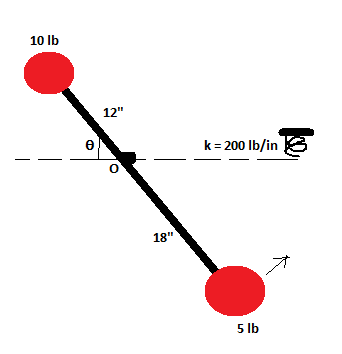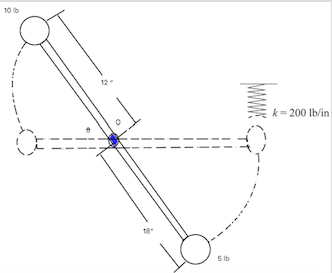# The light rod is pivoted at O and carries the 5 lb and 10 lb particles. If the rod is released...

## Question:

The light rod is pivoted at O and carries the 5 lb and 10 lb particles. If the rod is released from rest at {eq}\theta = 60^\circ {/eq} and swings in the vertical plane, calculate:

a. the velocity v of the 5 lb particle just before it hits the spring.

b. the maximum compression x of the spring. Assume that x is small so that the position of the rod when the spring is compressed is essentially horizontal.## Conservation of Energy:

Conservation of Energy states that an isolated system has a constant total energy.

{eq}U_1 + U_2 = K {/eq}

where {eq}K = \frac{1}{2}l \omega^2 {/eq} and {eq}U = mgh {/eq}

And we can compute for the speed using the formula:

{eq}v = \omega r {/eq}Part A.

Using Conservation of Energy, we can solve for the velocity of the 5 lb particle just before i hits the spring.

{eq}U_1 + U_2 = K {/eq}

where {eq}K = \frac{1}{2}l \omega^2 {/eq} and {eq}U = mgh {/eq}

{eq}m_1gh_1 + m_2 gh_2 = \frac{1}{2}l \omega^2 {/eq}

{eq}(10 \ lb)(12 \ in)(sin60^\circ) + (5 \ lb)(18 \ in)(sin60^\circ) = \frac{1}{2}(\frac{(10 \ lb)(12 \ in)^2 + (5 \ lb)(18 \ in)^2}{(32.17 \ ft/s^2)(12 \ in/ft)}) \omega^2 {/eq}

{eq}\omega^2 = \frac{(10 \ lb)(12 \ in)(sin60^\circ) + (5 \ lb)(-18 \ in)(sin60^\circ)}{\frac{1}{2}(\frac{(10 \ lb)(12 \ in)^2 + (5 \ lb)(18 \ in)^2}{(32.17 \ ft/s^2)(12 \ in/ft)})} {/eq}

{eq}\omega^2 = 2.56 \ rad/s {/eq}

{eq}v = \omega r {/eq}

{eq}v = (2.56 \ rad/s)(18 \ in) {/eq}

{eq}v = 46 \ m/s {/eq}

Part B.

Kinetic Energy is equal to Potential Energy of Spring

KE = SE

{eq}\frac{1}{2}l \omega^2 = \frac{1}{2} kx^2 {/eq}

{eq}\frac{1}{2}(\frac{(10 \ lb)(12 \ in)^2 + (5 \ lb)(18 \ in)^2}{(32.17 \ ft/s^2)(12 \ in/ft)})(46 \ m/s)^2 = \frac{1}{2} (200 \ lb/in) x^2 {/eq}

{eq}x^2 = \frac{\frac{1}{2}(\frac{(10 \ lb)(12 \ in)^2 + (5 \ lb)(18 \ in)^2}{(32.17 \ ft/s^2)(12 \ in/ft)})(46 \ m/s)^2}{\frac{1}{2} (200 \ lb/in)} {/eq}

{eq}x = 1.35 \ in{/eq}